Python 中最流行的十个标准库

2021/01/02 11:40

Python实战社群

Java实战社群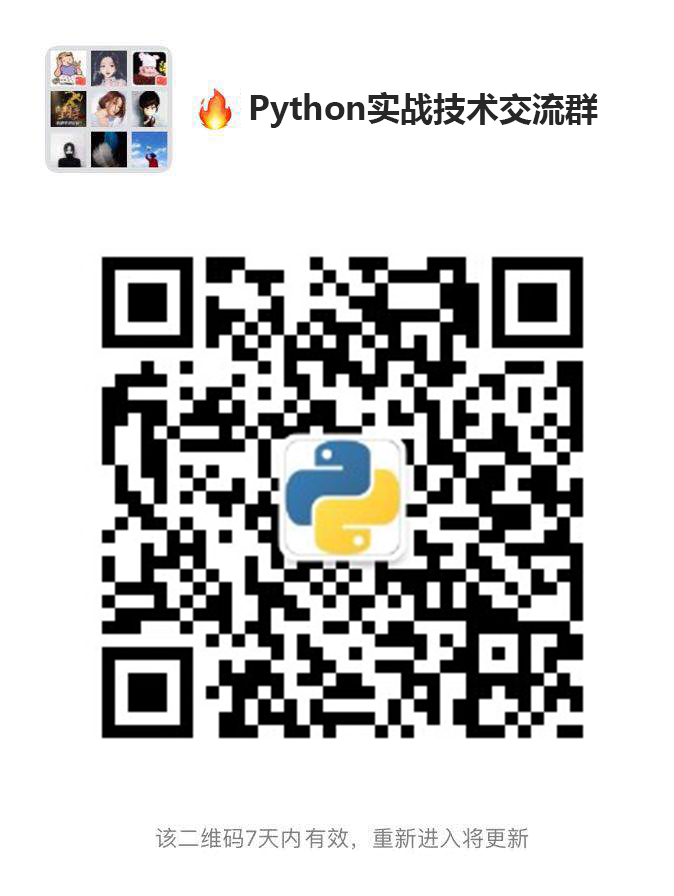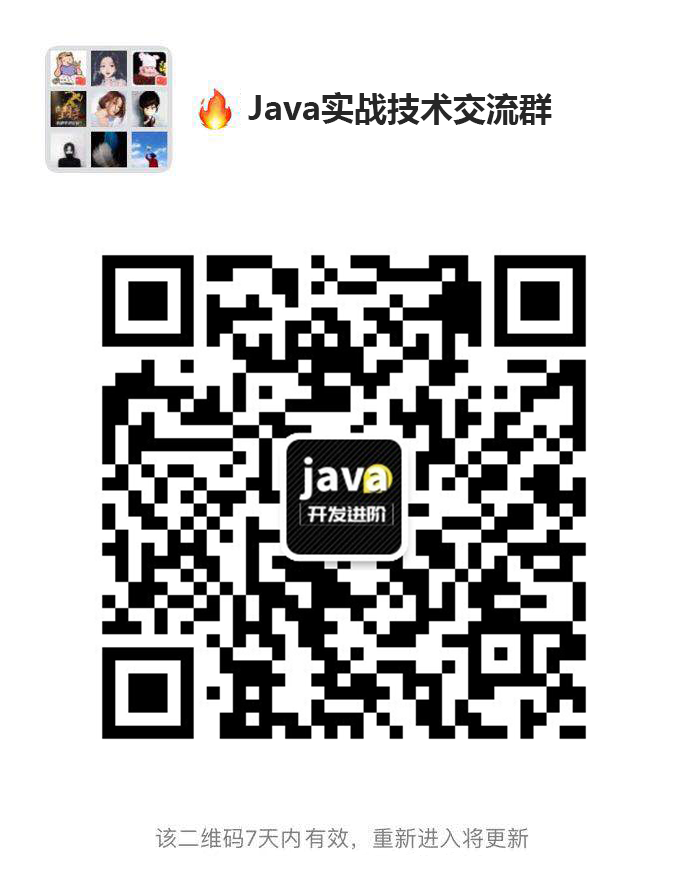Python 以其有用的库和包而著称，即使没有软件工程背景的人也能编程。本文基于 GitHub 仓库的示例数据集，探索流行的 Python 标准库。

Python 是当今人工智能和机器学习领域最流行的编程语言之一。Python 以其有用的库和包而著称，即使没有软件工程背景的人也能编程。

Python 拥有一组与 Python 语言一起分发的标准库，如 DateTime、math 或 Random。本文的目标是在 GitHub 的 Python 仓库中找到 10 个最有用的标准库。为实现我们的目标，我们研究了 GitHub 中不同的 Python 仓库，并收集了它们的旧库来回答这个问题。

pip install pydriller

#import libraries
import pydriller as pyd
from datetime import datetime
#period to collect data
dt1 = datetime(2019, 11, 1)
dt2 = datetime(2020, 11, 1)
#path of 5 Python repositories
path = ["https://github.com/django/django.git","https://github.com/pandas-dev/pandas.git",
"https://github.com/numpy/numpy","https://github.com/home-assistant/home-assistant.git",
"https://github.com/donnemartin/system-design-primer.git"]
#collecting a version of a source file before and after applying a commit
tf_source = pd.DataFrame(columns=['commit_ID', 'before_Commit', 'after_Commit'])
for commit in pyd.RepositoryMining(path_to_repo=path, since=dt1, to=dt2).traverse_commits():
for modified_file in commit.modifications:
if modified_file.filename.endswith(".py"):
tf_source = tf_source.append({'commit_ID': commit.hash,'before_Commit': modified_file.source_code_before,
'after_Commit': modified_file.source_code}, ignore_index=True)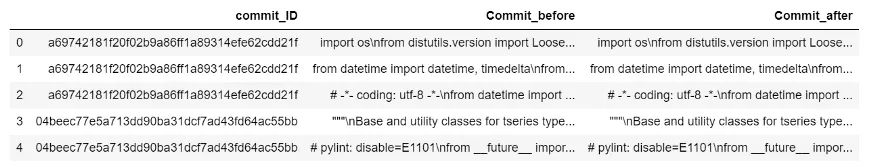1. 收集 Python 中所有可用的标准库的列表

2. 构建基于抽象语法书的函数来收集库名

#import libraries
import ast
import tokenize
#A class to walk trough AST and collect libraries name
class FuncParser(ast.NodeVisitor):
def visit_Import(self, node):
tempImpo = node.names
if(tempImpo != None):
listImpo = tempImpo
Impo = listImpo.name
if (Impo in api_name):
file_contents.append(Impo)
ast.NodeVisitor.generic_visit(self, node)
else:
ast.NodeVisitor.generic_visit(self, node)
def visit_ImportFrom(self, node):
module=node.module
if(module in api_name):
file_contents.append(module)
else:
ast.NodeVisitor.generic_visit(self, node)
def generic_visit(self, node):
ast.NodeVisitor.generic_visit(self, node)

import tokenize as tz
import assistant as ass

Module(body=[Import(names=[alias(name='tokenize', asname='tz')]), Import(names=[alias(name='assistant', asname='ass')])])

Python 仓库中基于 GitHub 提交的 10 个最流行标准库是什么

1. 提交提交之前收集库名列表

text_before=str(tf_source[‘Commit_before’](0))

bf_obj = FuncParser()
bf_tree = ast.parse(text_before)
file_contents = []
bf_obj.visit(bf_tree)
dtobj_before = pd.DataFrame(file_contents, columns=[‘token’])
tokens_before =pd.DataFrame(dtobj_before[‘token’].value_counts())

2. 提交提交之后收集库名列表

text_after=str(tf_source[‘Commit_after’](0))

aft_obj = FuncParser()
aft_tree = ast.parse(text_after)
file_contents = []
aft_obj.visit(aft_tree)
dtobj_after = pd.DataFrame(file_contents, columns=[‘token’])
tokens_after =pd.DataFrame(dtobj_after[‘token’].value_counts())

3. 找出这两个列表之间的差异

diff = tokens_after.subtract(tokens_before)
diff_token = diff[(diff.select_dtypes(include=[‘number’]) != 0).any(1)]
diff_token=diff_token.fillna(0)
diff_token= diff_token.abs()
diff_token = diff_token.reset_index()

4. 计算库的数量

py_lib={}
j=0
for j in range(0,len(diff_token)):
word = diff_token['index'](j).lower()
if word in py_lib:
py_lib[word]+=diff_token['token'](j)
else:
py_lib[word]=1
j+=

i=0
error=0
py_lib={}
for row in tf_source.iterrows():

#parsing the source file before applying commit i
if tf_source['Commit_before'](i) is not None:
try:
text_before=str(tf_source['Commit_before'](i))

bf_obj = FuncParser()
bf_tree = ast.parse(text_before)
file_contents = []
bf_obj.visit(bf_tree)
dtobj_before = pd.DataFrame(file_contents, columns=['token'])
tokens_before =pd.DataFrame(dtobj_before['token'].value_counts())

except:
error +=1
else:
file_contents = []
dtobj_before = pd.DataFrame(file_contents, columns=['token'])
tokens_before =pd.DataFrame(dtobj_before['token'].value_counts())

#parsing the source file after applying commit i
if tf_source['Commit_after'](i) is not None:
try:
text_after=str(tf_source['Commit_after'](i))

aft_obj = FuncParser()
aft_tree = ast.parse(text_after)
file_contents = []
aft_obj.visit(aft_tree)
dtobj_after = pd.DataFrame(file_contents, columns=['token'])
tokens_after =pd.DataFrame(dtobj_after['token'].value_counts())

except:
error +=1
else:
file_contents = []
dtobj_after = pd.DataFrame(file_contents, columns=['token'])
tokens_after =pd.DataFrame(dtobj_after['token'].value_counts())

#calculating the differences between two list tokens_before and tokens_after
diff = tokens_after.subtract(tokens_before)
diff_token = diff[(diff.select_dtypes(include=['number']) != 0).any(1)]
diff_token=diff_token.fillna(0)
diff_token= diff_token.abs()
diff_token = diff_token.reset_index()

# counting the numer of each libraries which are added or removed by commit i
j=0
for j in range(0,len(diff_token)):
word = diff_token['index'](j).lower()
if word in py_lib:
py_lib[word]+=diff_token['token'](j)
else:
py_lib[word]=1
j+=1

i+=1

from operator import itemgetter
d=sorted(py_lib.items(), key=itemgetter(1),reverse=True)[:10]
[('warnings', 96.0),
('sys', 73.0),
('datetime', 28.0),
('test', 27.0),
('os', 22.0),
('collections', 18.0),
('io', 16.0),
('gc', 10.0),
('functools', 9.0),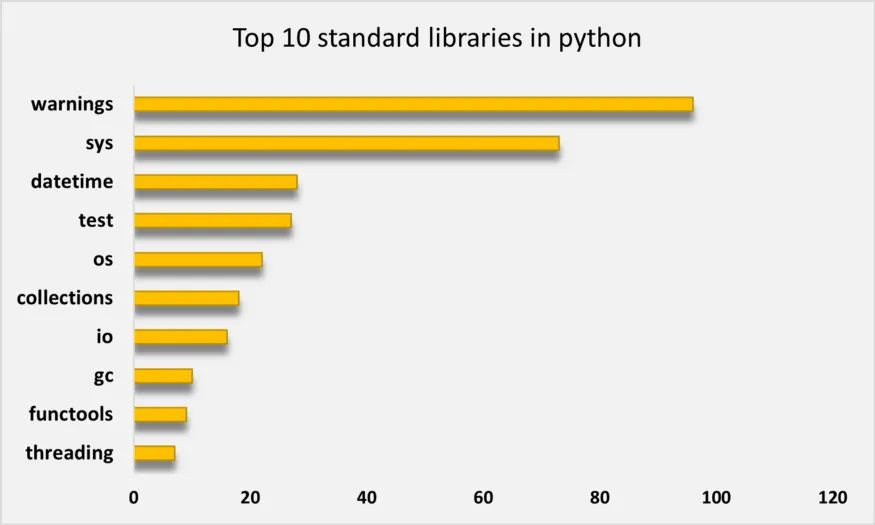import matplotlib.pyplot as plt
from wordcloud import WordCloud
wordcloud = WordCloud(background_color='black',max_font_size = 50)
wordcloud.generate_from_frequencies(frequencies=py_lib)
plt.figure(figsize=(8,6))
plt.imshow(wordcloud, interpolation="bilinear")
plt.axis("off")
plt.show()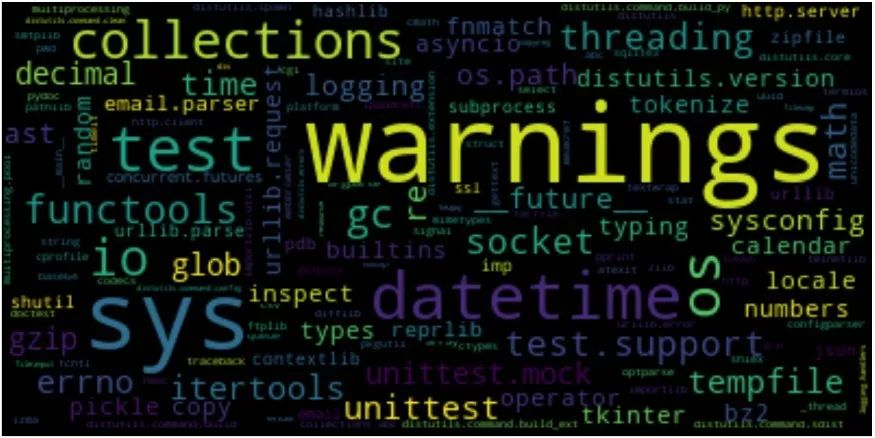https://towardsdatascience.com/what-are-the-10-most-popular-standard-libraries-in-python-359defb104d6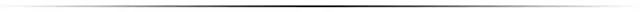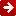看电影前一定要检查一下域名是不是HTTPS的有个大神级女朋友是什么体验世界上五个最不务正业的科学家！魂斗罗只有128KB为何可以实现那么长的剧情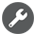0
0 收藏

作者的其它热门文章0 评论
0 收藏
0